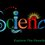# Cube Roots

In this note I am going to tell you the world's fastest method to find the cube root of any $6-digit number$. It takes nearly 2-3 mins to find the cube root but using this method you can find it in just 2 seconds.

For this trick you must know the cubes from numbers $1 to 10$ and you will observe an interesting property.

$1^3 = 1$ ---- Cubes ending in 1 have cube roots also ending in 1.

$2^3 = 8$ ---- Cubes ending in 8 have cube roots ending in 2.

$3^3 = 27$ ---- Cubes ending in 7 have cube roots ending in 3.

$4^3 = 64$ ---- Cubes ending in 4 have cube roots ending in 4.

$5^3 = 125$ ---- Cubes ending in 5 have cube roots ending in 5.

$6^3 = 216$ ---- Cubes ending in 6 have cube roots ending in 6.

$7^3 = 343$ ---- Cubes ending in 3 have cube roots ending in 7.

$8^3 = 512$ ---- Cubes ending in 2 have cube roots ending in 8.

$9^3 = 729$ ---- Cubes ending in 9 have cube roots ending in 9.

$10^3 = 1000$ ---- Cubes ending in 0 have cube roots ending in 0.

Now you will observe an interesting property that cubes ending in 3 have cube roots ending in 7 and cubes ending in 7 have cube roots ending in 3. Another interesting property is that cubes ending in 2 have cube roots ending in 8 and cubes ending in 8 have cube roots ending in 2.

Now we will take an example:-

Find the cube root of 1728 = Which means the answer is ending in 2.

Now follow the next step to find the number in the tens place.

Make groups of 3 starting from units place = $1 , 728 ,$

The first group of 728 is done and the answer for that group is 2. Now take the next group which is 1. Find the smallest cube which you can subtract form 1. The smallest cube you can subtract is 1. So, the answer is $12.$

Note that I am not actually subtracting but I am just observing.

Lets take another example:-

Find the cube root of 614125.

= $614 , 125 ,$ ---- { The answer is ending in 5 }

= The greatest cube you can subtract from 614 is 512 which is a cube root of 8.

So, the cube root of $614125$ is $85.$5 years, 7 months ago

This discussion board is a place to discuss our Daily Challenges and the math and science related to those challenges. Explanations are more than just a solution — they should explain the steps and thinking strategies that you used to obtain the solution. Comments should further the discussion of math and science.

When posting on Brilliant:

• Use the emojis to react to an explanation, whether you're congratulating a job well done , or just really confused .
• Ask specific questions about the challenge or the steps in somebody's explanation. Well-posed questions can add a lot to the discussion, but posting "I don't understand!" doesn't help anyone.
• Try to contribute something new to the discussion, whether it is an extension, generalization or other idea related to the challenge.

MarkdownAppears as
*italics* or _italics_ italics
**bold** or __bold__ bold
- bulleted- list
• bulleted
• list
1. numbered2. list
1. numbered
2. list
Note: you must add a full line of space before and after lists for them to show up correctly
paragraph 1paragraph 2

paragraph 1

paragraph 2

[example link](https://brilliant.org)example link
> This is a quote
This is a quote
    # I indented these lines
# 4 spaces, and now they show
# up as a code block.

print "hello world"
# I indented these lines
# 4 spaces, and now they show
# up as a code block.

print "hello world"
MathAppears as
Remember to wrap math in $$ ... $$ or $ ... $ to ensure proper formatting.
2 \times 3 $2 \times 3$
2^{34} $2^{34}$
a_{i-1} $a_{i-1}$
\frac{2}{3} $\frac{2}{3}$
\sqrt{2} $\sqrt{2}$
\sum_{i=1}^3 $\sum_{i=1}^3$
\sin \theta $\sin \theta$
\boxed{123} $\boxed{123}$

Sort by:

Fantastic method bro.. Well said ....

- 5 years, 7 months ago

Well I know how to do the world's fastest method for 5th roots. BAM! :D

- 5 years, 7 months ago

- 5 years, 7 months ago

Remember 1, 32, 243, 1024, 3125, 7776, 16807, 32768 and 59049. Notice that the units digit for each of these numbers are the units digit for their 5th roots. This is for all integer answers to the roots such that the integer is 1 or 2 digits. The rest you can follow from the note.

- 5 years, 7 months ago

Well said

- 5 years, 7 months ago